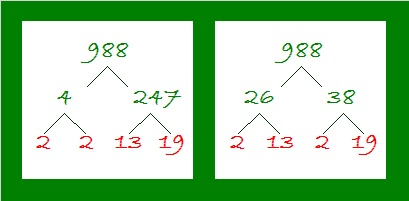# 988 Christmas Factor Tree

To solve a level 3 puzzle, find two clues in a single row or column. They will help you know what factors to put in the top cell of the first column and two other cells in either the first column or top row. You’ll then be able to work your way down the puzzle by finding the factors of each clue in turn in the most ideal order. The clues below a current clue can still affect the logic of that clue, as you should discover with this puzzle.Print the puzzles or type the solution in this excel file: 10-factors-986-992

Here’s a little about the number 988:

It is divisible by 4 because 88 is divisible by 4. Here are a couple of its factor trees that are nicely well-balanced:988 is the sum of these four consecutive prime numbers:
239 + 241 + 251 + 257 = 988

988 is also the hypotenuse of a Pythagorean triple:
380-912-988 which is (5-12-13) times 76

• 988 is a composite number.
• Prime factorization: 988 = 2 × 2 × 13 × 19, which can be written 988 = 2² × 13 × 19
• The exponents in the prime factorization are 2, 1, and 1. Adding one to each and multiplying we get (2 + 1)(1 + 1)(1 + 1) = 3 × 2 × 2 = 12. Therefore 988 has exactly 12 factors.
• Factors of 988: 1, 2, 4, 13, 19, 26, 38, 52, 76, 247, 494, 988
• Factor pairs: 988 = 1 × 988, 2 × 494, 4 × 247, 13 × 76, 19 × 52, or 26 × 38
• Taking the factor pair with the largest square number factor, we get √988 = (√4)(√247) = 2√247 ≈ 31.432467Now, what do you imagine is the total number of triangles of any size in the graphic below? You guessed it, 988.This site uses Akismet to reduce spam. Learn how your comment data is processed.